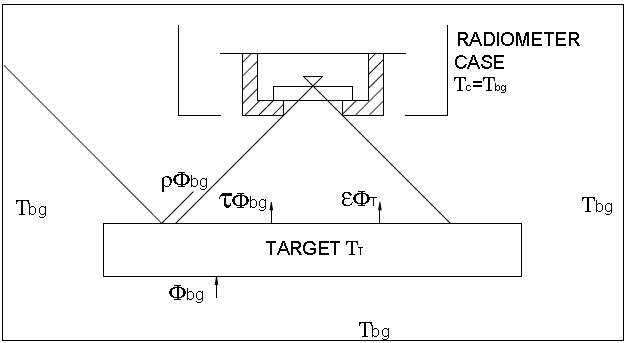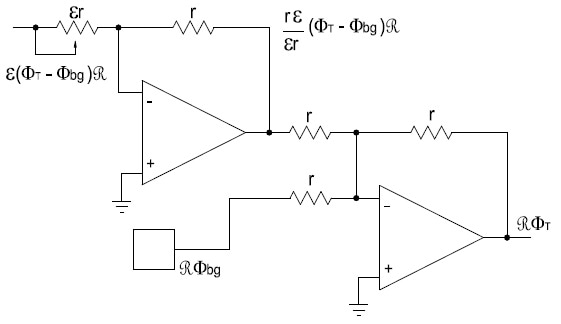# Correcting Emissivity for a Radiometer

The radiant power received by a radiometer is both a function of the quantified target temperature and the optical properties of its surface. These properties comprise of reflectance ρ, absorptance a, and transmittance τ. For a Lambertian type surface, these properties are related by Kirchoff’s law:

 a + ρ + τ = 1 (1)

## Emissivity Correction for a Radiometer

For the majority of surfaces, it can be assumed that ε = a where ε is the specimen’s emissive power and by definition emissivity (ε) is the ratio of energy discharged from the target and that of a blackbody at the same temperature as the target. The objective of Figure 1 is to demonstrate the radiative balance between a radiometer, a target and the environmental ambient background.The total radiant power Φt incident on the active junctions of the thermopile detector is:

 ΦTOT = εΦT + ρΦbg + τΦbg (2)

Using the Kirchoff’s law, this equation can be written as:

 ΦTOT = εΦT + (1-ε) + Φbg (3)

The active junctions of the thermopile radiate the energy which is Φc and equals Φw when the radiometer case is the same temperature as the ambient background (bg). Now, the energy balance for the active junction of the thermopile is:

 Φd = ΦTOT - Φc = εΦT + (1-ε) Φbg - Φc (4)

For the special conditions of the radiometer case and walls being isothermal:

 Φd = εΦT + εΦbg (5)

The voltage Vd produced by the thermopile is:

 Vd = ℜΦd = εℜ(ΦT - Φbg) (6)

Where ℜ represents the coefficient of responsivity of thermopile in watt/volts.

Given that emissivity enters into the calculation as a multiplication term, a linear amplifier with gain of 1/ε can be used to cancel its effect. Figure 2 shows an emissivity correction circuit.Figure 2. Emissivity Correction Circuit.

This computation method solves directly for ℜΦT, which is in proportion to target temperature. In addition, the correction is linear, and hence, a linear variable resistor with a turns counting dial may be directly calibrated in emissivity.

## Conclusion

This article shows that when a temperature radiometer is employed in an isothermal environment, a basic computation circuit using linear amplifiers can be developed to correct the target emissivity.This information has been sourced, reviewed and adapted from materials provided by Dexter Research Center, Inc.

## Citations

• APA

Dexter Research Center, Inc.. (2019, December 24). Correcting Emissivity for a Radiometer. AZoSensors. Retrieved on November 28, 2020 from https://www.azosensors.com/article.aspx?ArticleID=906.

• MLA

Dexter Research Center, Inc.. "Correcting Emissivity for a Radiometer". AZoSensors. 28 November 2020. <https://www.azosensors.com/article.aspx?ArticleID=906>.

• Chicago

Dexter Research Center, Inc.. "Correcting Emissivity for a Radiometer". AZoSensors. https://www.azosensors.com/article.aspx?ArticleID=906. (accessed November 28, 2020).

• Harvard

Dexter Research Center, Inc.. 2019. Correcting Emissivity for a Radiometer. AZoSensors, viewed 28 November 2020, https://www.azosensors.com/article.aspx?ArticleID=906.